# TCO19 Marathon Match 106 - Marathon Match 106

## Key Information

Register
Submit
Competition Timeline

Timezone:Etc/UTC

Registration

Starts

Jan 05, 2019

02:00

Ends

Jan 15, 2019

02:00

Submission

Starts

Jan 05, 2019

02:00

Ends

Jan 15, 2019

02:00

Winners Announced

Jan 15, 2019

02:00

## Challenge Overview

### Problem Statement

You are given an image, and you want to create a stained glass panel from it. To do this, you need to find the best way to approximate the image with an arrangement of colored pieces of glass. Your colored glass supplier is not a huge fan of delivering you a thousand small pieces of slightly different colors, so he'll charge you for the number of colors you use.

To make the window nice and sturdy, you decide to approximate the image as a Voronoi diagram of a set of seed points. Each piece of glass is formed by all pixels that are closer to the corresponding seed point than to any other seed point; in case of a tie, the pixel belongs to the piece with the smallest index. The pixels in each piece of glass are assigned the same color. Here is an example of such a diagram, with seed points marked with black squares.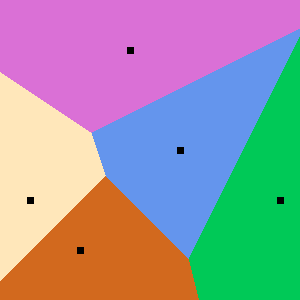An animated gif showing several different stained glass images for seed 2 can be found here.

### Implementation

Your code should implement a single method create(int H, int[] pixels, int N).
• H is the height of the image. You can get the width of the image W as pixels.size() / H.
• pixels gives you the image itself: pixels[R*W+C] describes the color of pixel in row R and column C, encoded as an integer with 8 bits per color component in RGB order. You can decode color components as follows:
`int R = (pixel >> 16), G = (pixel >> 8) & 0xff, B = pixel & 0xff`
• N is the maximal number of pieces of glass you can use. You don't have to use all of them, but you don't get extra points for using less than N.
The method should return a int[] describing the glass pieces you want to use. Piece i should be described with three numbers:
• ret[3*i] is the row coordinate of the pixel which contains the seed point of the piece (must be between -1000 and H+999, inclusive).
• ret[3*i+1] is the column coordinate of the pixel which contains the seed point of the piece (must be between -1000 and W+999, inclusive).
• ret[3*i+2] is the color of the glass used in the piece (in the same format as the colors in the pixels array, must be between 0x000000 and 0xFFFFFF, inclusive).
Note that you can't have two seed points with the same coordinates.

### Scoring

For each test case we will calculate your raw score. If your solution produced an invalid return (invalid description of the glass pieces or too many of them), raw score for this test case will be -1. Otherwise, the score will be the sum of absolute values of component-wise differences of colors of each pixel in the original image and in your approximation, multiplied by (1 + (number of distinct colors used)/(number of pieces used))^2.

Your overall score will be calculated in the following way: for each test case where your score is not -1, you get 1 point for each competitor you beat on this test case (i.e., your score on a test case is less than this competitor's score) and 0.5 points for each competitor you tie with (a tie with yourself is not counted); finally, the sum of points is divided by (the number of competitors - 1), then multiplied by 1000000 and divided by the number of test cases.

Finally, your total score is equal to the arithmetic average of normalized scores on all test cases, multiplied by 1,000,000.

### Tools

An offline tester is available here. You can use it to test/debug your solution locally. You can also check its source code for exact implementation of test case generation and score calculation. That page also contains example images, links to useful information and sample solutions in several languages.

### Definition

 Class: StainedGlass Method: create Parameters: int, int[], int Returns: int[] Method signature: int[] create(int H, int[] pixels, int N) (be sure your method is public)

### Notes

-N is chosen randomly between 20 and 1000.
-Images used in tests were downloaded from random image at Wikipedia. Images which were not primarily photographic or photo-realistic, such as line drawings or photos of paintings, were discarded. Each dimension of each image will be between 250 and 800 pixels.
-The time limit is 20 seconds per test case (this includes only the time spent in your code). The memory limit is 1024 megabytes.
-There is no explicit code size limit. The implicit source code size limit is around 1 MB (it is not advisable to submit codes of size close to that or larger). Once your code is compiled, the binary size should not exceed 1 MB.
-The compilation time limit is 30 seconds. You can find information about compilers that we use and compilation options here.
-There are 10 example test cases and 100 full submission (provisional) test cases. There will be 1000 test cases in the final testing.
-The match is rated.

### Examples

0)

 `"1"`
```Returns: "seed = 1
N = 20
"```1)

 `"2"`
```Returns: "seed = 2
N = 1000
"```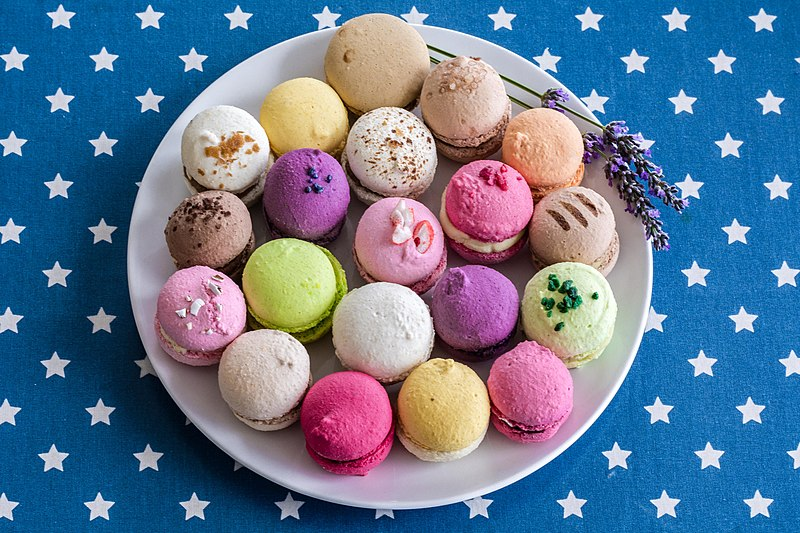2)

 `"3"`
```Returns: "seed = 3
N = 555
"```3)

 `"4"`
```Returns: "seed = 4
N = 518
"```4)

 `"5"`
```Returns: "seed = 5
N = 970
"```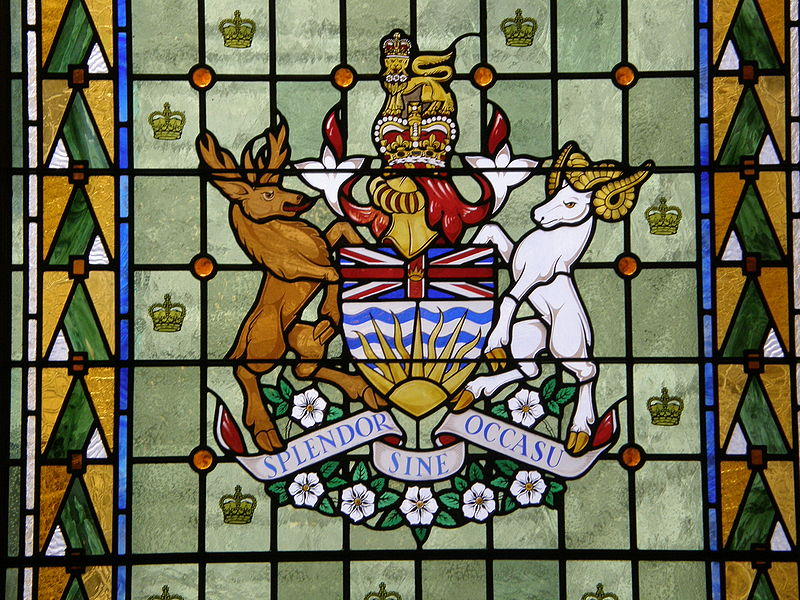5)

 `"6"`
```Returns: "seed = 6
N = 728
"```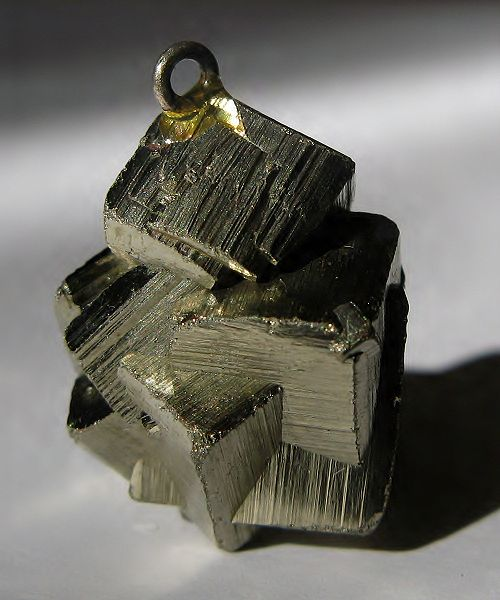6)

 `"7"`
```Returns: "seed = 7
N = 152
"```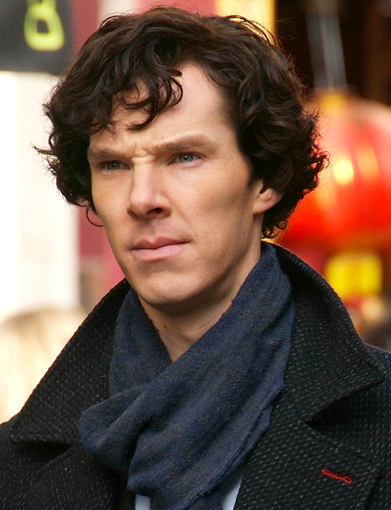7)

 `"8"`
```Returns: "seed = 8
N = 294
"```8)

 `"9"`
```Returns: "seed = 9
N = 552
"```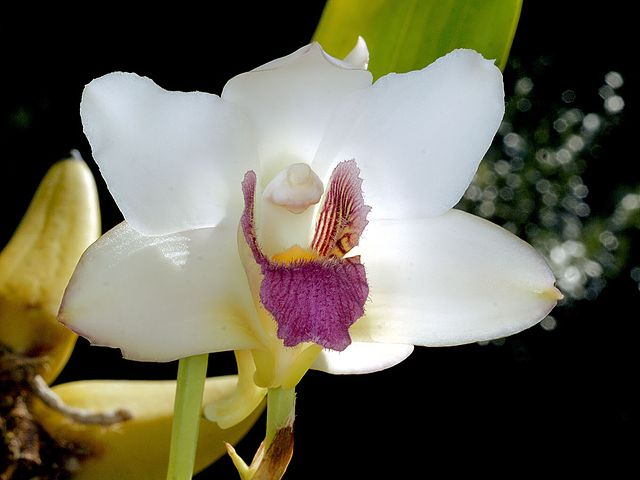9)

 `"10"`
```Returns: "seed = 10
N = 474
"```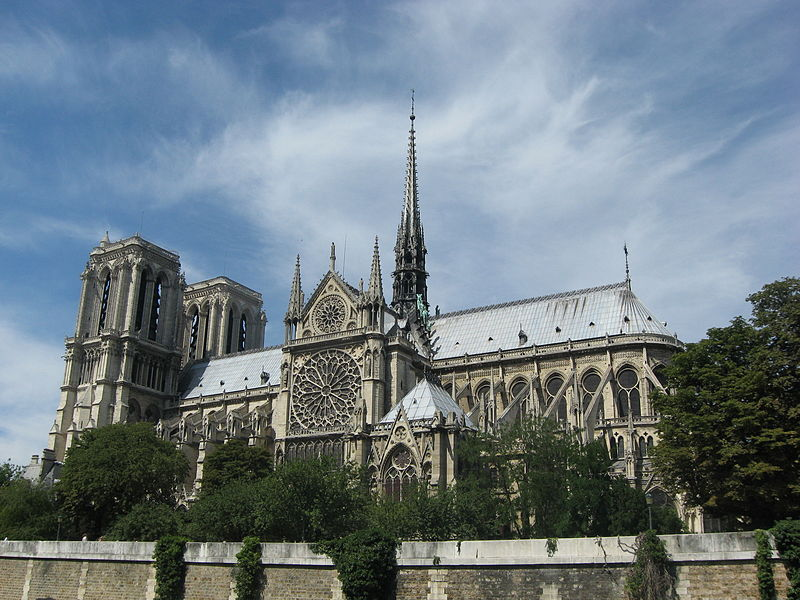This problem statement is the exclusive and proprietary property of TopCoder, Inc. Any unauthorized use or reproduction of this information without the prior written consent of TopCoder, Inc. is strictly prohibited. (c)2020, TopCoder, Inc. All rights reserved.

## LEARN:

Topcoder Challenges Explained